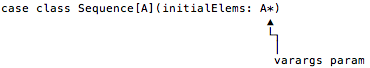# How To Make Sequence Work as a Single Generator in a `for` Expression

This is a page from my book, Functional Programming, Simplified

Getting `Sequence` to work as a generator in a simple `for` loop was cool, but does adding `foreach` let `Sequence` also work when I add `yield`? Let’s see.

When I paste this code into the REPL:

``````val ints = Sequence(1,2,3)

for {
i <- ints
} yield i*2``````

I see this error message:

``````scala> for {
|     i <- ints
| } yield i*2
<console>:15: error: value map is not a member of Sequence[Int]
i <- ints
^``````

Sadly, `Sequence` won’t currently work with `for`/`yield`, but again the REPL tells us why:

``error: value map is not a member of Sequence[Int]``

That error tells us that `Sequence` needs a `map` method for this to work. Great — let’s create one.

## Adding a `map` method to Sequence

Again I’m going to cheat to create a simple solution, this time using `ArrayBuffer`’s `map` method inside `Sequence`’s `map` method:

``````def map[B](f: A => B): Sequence[B] = {
val abMap: ArrayBuffer[B] = elems.map(f)
Sequence(abMap: _*)
}``````

This `map` method does the following:

• It takes a function input parameter that transforms a type `A` to a type `B`.
• When it’s finished, `map` returns a `Sequence[B]`.
• In the first line of the function I show `abMap: ArrayBuffer[B]` to be clear that `elems.map(f)` returns an `ArrayBuffer`. As usual, showing the type isn’t necessary, but I think it helps to make this step clear.
• In the second line inside the function I use the `:_*` syntax to create a new `Sequence` and return it.

### About the `:_*` syntax

If you haven’t seen the `abMap: _*` syntax before, the `:_*` part of the code is a way to adapt a collection to work with a varargs parameter. Recall that the `Sequence` constructor is defined to take a varags parameter:For more information on this syntax, see my information on Scala’s missing splat operator.

## The complete Sequence class

This is what the `Sequence` class looks like when I add the `map` method to it:

``````case class Sequence[A](initialElems: A*) {

private val elems = scala.collection.mutable.ArrayBuffer[A]()

// initialize
elems ++= initialElems

def map[B](f: A => B): Sequence[B] = {
val abMap = elems.map(f)
new Sequence(abMap: _*)
}

def foreach(block: A => Unit): Unit = {
elems.foreach(block)
}

}``````

## Does for/yield work now?

Now when I go back and try to use the `for`/`yield` expression I showed earlier, I find that it compiles and runs just fine:

``````scala> val ints = Sequence(1,2,3)
ints: Sequence[Int] = Sequence(WrappedArray(1, 2, 3))

scala> for {
|     i <- ints
| } yield i*2
res0: Sequence[Int] = Sequence(ArrayBuffer(2, 4, 6))``````

## An important point

One point I need to make clear is that this `for`/`yield` expression works solely because of the `map` method; it has nothing to do with the `foreach` method.

You can demonstrate this in at least two ways. First, if you remove the `foreach` method from the `Sequence` class you’ll see that this `for` expression still works.

Second, if you create a little test class with this code in it, and then compile it with `scalac -Xprint:parse`, you’ll see that the Scala compiler converts this `for` expression:

``````for {
i <- ints
} yield i*2``````

into this `map` expression:

``ints.map(((i) => i.\$times(2)))``

To be very clear, creating a `foreach` in `Sequence` enables this `for` loop:

``for (i <- ints) println(i)``

and defining a `map` method in `Sequence` enables this `for` expression:

``````for {
i <- ints
} yield i*2``````

## Summary

I can summarize what I accomplished in this lesson and the previous lesson with these lines of code:

``````// (1) works because `foreach` is defined
for (p <- peeps) println(p)

// (2) `yield` works because `map` is defined
val res: Sequence[Int] = for {
i <- ints
} yield i * 2
res.foreach(println)  // verify the result``````

## What’s next?

This is a good start. Next up, I’ll modify `Sequence` so I can use it with filtering clauses in `for` expressions.